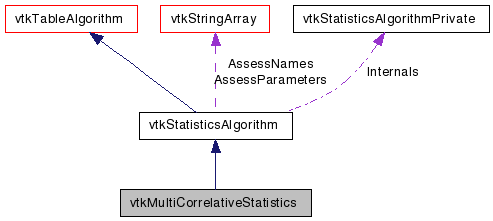# vtkMultiCorrelativeStatistics Class Reference

`#include <vtkMultiCorrelativeStatistics.h>`

Inheritance diagram for vtkMultiCorrelativeStatistics:[legend]
Collaboration diagram for vtkMultiCorrelativeStatistics:[legend]

## Detailed Description

A class for linear correlation.

Given a selection of sets of columns of interest, this class provides the following functionalities, depending on the execution mode it is executed in: Learn: calculates means, unbiased variance and covariance estimators of column pairs coefficient. More precisely, Learn calculates the averages and centered variance/covariance sums; if `finalize` is set to true (default), the final statistics are calculated. The output metadata on port OUTPUT_MODEL is a multiblock dataset containing at a minimum one vtkTable holding the raw sums in a sparse matrix style. If finalize is true, then one additional vtkTable will be present for each requested set of column correlations. These additional tables contain column averages, the upper triangular portion of the covariance matrix (in the upper right hand portion of the table) and the Cholesky decomposition of the covariance matrix (in the lower portion of the table beneath the covariance triangle). The leftmost column will be a vector of column averages. The last entry in the column averages vector is the number of samples. As an example, consider a request for a 3-column correlation with columns named ColA, ColB, and ColC. The resulting table will look like this:

```      Column  |Mean     |ColA     |ColB     |ColC
--------+---------+---------+---------+---------
ColA    |avg(A)   |cov(A,A) |cov(A,B) |cov(A,C)
ColB    |avg(B)   |chol(1,1)|cov(B,B) |cov(B,C)
ColC    |avg(C)   |chol(2,1)|chol(2,2)|cov(C,C)
Cholesky|length(A)|chol(3,1)|chol(3,2)|chol(3,3)
```
Assess: given a set of results matrices as specified above in input port INPUT_MODEL and tabular data on input port INPUT_DATA that contains column names matching those of the tables on input port INPUT_MODEL, the assess mode computes the relative deviation of each observation in port INPUT_DATA's table according to the linear correlations implied by each table in port INPUT_MODEL.

Thanks:
Thanks to Philippe Pebay, Jackson Mayo, and David Thompson of Sandia National Laboratories for implementing this class.
Examples:
vtkMultiCorrelativeStatistics (Examples)
Tests:
vtkMultiCorrelativeStatistics (Tests)

Definition at line 76 of file vtkMultiCorrelativeStatistics.h.

## Public Types

typedef vtkStatisticsAlgorithm Superclass

## Public Member Functions

virtual const char * GetClassName ()
virtual int IsA (const char *type)
virtual void PrintSelf (ostream &os, vtkIndent indent)
virtual void Aggregate (vtkDataObjectCollection *, vtkMultiBlockDataSet *)

## Static Public Member Functions

static int IsTypeOf (const char *type)
static
vtkMultiCorrelativeStatistics
SafeDownCast (vtkObject *o)
static
vtkMultiCorrelativeStatistics
New ()

## Protected Member Functions

vtkMultiCorrelativeStatistics ()
~vtkMultiCorrelativeStatistics ()
virtual void Derive (vtkMultiBlockDataSet *)
virtual void Learn (vtkTable *inData, vtkTable *inParameters, vtkMultiBlockDataSet *outMeta)
virtual void Assess (vtkTable *, vtkMultiBlockDataSet *, vtkTable *)
virtual void Test (vtkTable *, vtkMultiBlockDataSet *, vtkTable *)
virtual void SelectAssessFunctor (vtkTable *inData, vtkDataObject *inMeta, vtkStringArray *rowNames, AssessFunctor *&dfunc)

## Member Typedef Documentation

Reimplemented from vtkStatisticsAlgorithm.

Reimplemented in vtkPCAStatistics, vtkPMultiCorrelativeStatistics, and vtkPPCAStatistics.

Definition at line 79 of file vtkMultiCorrelativeStatistics.h.

## Constructor & Destructor Documentation

 vtkMultiCorrelativeStatistics::vtkMultiCorrelativeStatistics ( ) ` [protected]`

 vtkMultiCorrelativeStatistics::~vtkMultiCorrelativeStatistics ( ) ` [protected]`

## Member Function Documentation

 virtual const char* vtkMultiCorrelativeStatistics::GetClassName ( ) ` [virtual]`

 static int vtkMultiCorrelativeStatistics::IsTypeOf ( const char * name ) ` [static]`

Return 1 if this class type is the same type of (or a subclass of) the named class. Returns 0 otherwise. This method works in combination with vtkTypeMacro found in vtkSetGet.h.

Reimplemented from vtkStatisticsAlgorithm.

Reimplemented in vtkPCAStatistics, vtkPMultiCorrelativeStatistics, and vtkPPCAStatistics.

 virtual int vtkMultiCorrelativeStatistics::IsA ( const char * name ) ` [virtual]`

Return 1 if this class is the same type of (or a subclass of) the named class. Returns 0 otherwise. This method works in combination with vtkTypeMacro found in vtkSetGet.h.

Reimplemented from vtkStatisticsAlgorithm.

Reimplemented in vtkPCAStatistics, vtkPMultiCorrelativeStatistics, and vtkPPCAStatistics.

 static vtkMultiCorrelativeStatistics* vtkMultiCorrelativeStatistics::SafeDownCast ( vtkObject * o ) ` [static]`

 virtual void vtkMultiCorrelativeStatistics::PrintSelf ( ostream & os, vtkIndent indent ) ` [virtual]`

Methods invoked by print to print information about the object including superclasses. Typically not called by the user (use Print() instead) but used in the hierarchical print process to combine the output of several classes.

Reimplemented from vtkStatisticsAlgorithm.

Reimplemented in vtkPCAStatistics, vtkPMultiCorrelativeStatistics, and vtkPPCAStatistics.

 static vtkMultiCorrelativeStatistics* vtkMultiCorrelativeStatistics::New ( ) ` [static]`

Create an object with Debug turned off, modified time initialized to zero, and reference counting on.

Reimplemented from vtkTableAlgorithm.

Reimplemented in vtkPCAStatistics, vtkPMultiCorrelativeStatistics, and vtkPPCAStatistics.

 virtual void vtkMultiCorrelativeStatistics::Aggregate ( vtkDataObjectCollection * , vtkMultiBlockDataSet * ) ` [virtual]`

Given a collection of models, calculate aggregate model

Implements vtkStatisticsAlgorithm.

 virtual void vtkMultiCorrelativeStatistics::Learn ( vtkTable * inData, vtkTable * inParameters, vtkMultiBlockDataSet * outMeta ) ` [protected, virtual]`

Execute the calculations required by the Learn option.

Implements vtkStatisticsAlgorithm.

Reimplemented in vtkPMultiCorrelativeStatistics, and vtkPPCAStatistics.

 virtual void vtkMultiCorrelativeStatistics::Derive ( vtkMultiBlockDataSet * ) ` [protected, virtual]`

Execute the calculations required by the Derive option.

Implements vtkStatisticsAlgorithm.

Reimplemented in vtkPCAStatistics.

 virtual void vtkMultiCorrelativeStatistics::Assess ( vtkTable * , vtkMultiBlockDataSet * , vtkTable * ) ` [protected, virtual]`

Execute the calculations required by the Assess option.

Implements vtkStatisticsAlgorithm.

Reimplemented in vtkPCAStatistics.

 virtual void vtkMultiCorrelativeStatistics::Test ( vtkTable * , vtkMultiBlockDataSet * , vtkTable * ) ` [inline, protected, virtual]`

Execute the calculations required by the Test option.

Implements vtkStatisticsAlgorithm.

Reimplemented in vtkPCAStatistics.

Definition at line 112 of file vtkMultiCorrelativeStatistics.h.

 virtual void vtkMultiCorrelativeStatistics::SelectAssessFunctor ( vtkTable * inData, vtkDataObject * inMeta, vtkStringArray * rowNames, AssessFunctor *& dfunc ) ` [protected, virtual]`

Provide the appropriate assessment functor.

Implements vtkStatisticsAlgorithm.

Reimplemented in vtkPCAStatistics.

The documentation for this class was generated from the following file:

Generated on Wed Aug 24 11:49:12 2011 for VTK by1.5.6